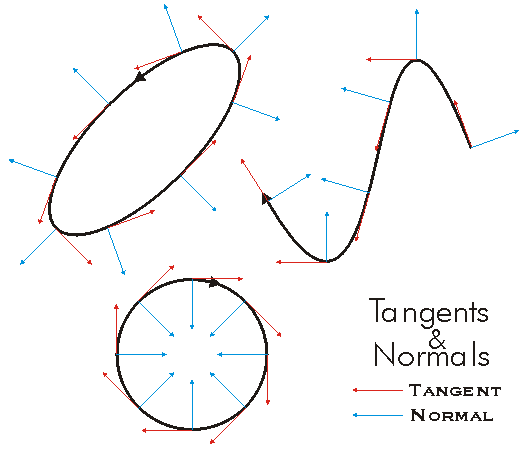This document is archived and information here might be outdated.  Recommended version.

ICurve.QueryTangent Method (ArcObjects .NET 10.8 SDK)
 ArcObjects Help for .NET developers > ArcObjects Help for .NET developers > ArcObjects namespaces > Geometry > ESRI.ArcGIS.Geometry > Interfaces > IC > ICurve Interface > ICurve.QueryTangent Method
 ArcGIS Developer Help

# ICurve.QueryTangent Method

Constructs a line tangent to a curve from a point at a specified distance along the curve.

```[Visual Basic .NET]
Public Sub QueryTangent ( _
ByVal extension As esriSegmentExtension, _
ByVal DistanceAlongCurve As Double, _
ByVal asRatio As Boolean, _
ByVal Length As Double, _
ByVal tangent As ILine _
)
```
```[C#]
public void QueryTangent (
esriSegmentExtension extension,
double DistanceAlongCurve,
bool asRatio,
double Length,
ILine tangent
);
```
```[C++]
HRESULT QueryTangent(
esriSegmentExtension extension,
double DistanceAlongCurve,
VARIANT_BOOL asRatio,
double Length,
ILine* tangent
);
```
```[C++]
Parameters
extension
extension is a parameter of type esriSegmentExtension
DistanceAlongCurve
DistanceAlongCurve is a parameter of type double
asRatio
asRatio is a parameter of type bool
Length
Length is a parameter of type double
tangent
tangent is a parameter of type ILine*
```

#### Product Availability

Available with ArcGIS Engine, ArcGIS Desktop, and ArcGIS Server.

#### Description

Given a distance along the curve specified either as a ratio of the length or as a specific fixed distance, QueryTangent returns the Line tangent to the Point.  The length and method of tangential extension of the tangent line are given by the user.  The method of tangential extension determines the direction of the tangent line as though it were being extended at a From point or a To point.

#### Remarks